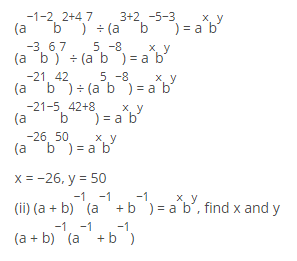Deepak Scored 45->99%ile with Bounce Back Crack Course. You can do it too!

# If a and b are different positive primes such that

Question:

If a and b are different positive primes such that

(i) $\left(\frac{a^{-1} b^{2}}{a^{2} b^{-4}}\right)^{7} \div\left(\frac{a^{3} b^{-5}}{a^{-2} b^{3}}\right)=a^{x} b^{y}$ find $x$ and $y$

(ii) $(a+b)^{-1}\left(a^{-1}+b^{-1}\right)=a^{x} b^{y}$, find $x$ and $y$

Solution:

(i) $\left(\frac{a^{-1} b^{2}}{a^{2} b^{-4}}\right)^{7} \div\left(\frac{a^{3} b^{-5}}{a^{-2} b^{3}}\right)=a^{x} b^{y}$ find $x$ and $y$

$\left(\frac{a^{-1} b^{2}}{a^{2} b^{-4}}\right)^{7} \div\left(\frac{a^{3} b^{-5}}{a^{-2} b^{3}}\right)=a^{x} b^{y}$$=\left(\frac{1}{a+b}\right)\left(\frac{1}{a}+\frac{1}{b}\right)$

$=\left(\frac{1}{a+b}\right)\left(\frac{b+a}{a b}\right)$

$=1 / a b$

$=(a b)^{-1}=a^{-1} b^{-1}$

By equating exponents

x = −1, y = −1

Therefore $x+y+2=-1-1+2=0$